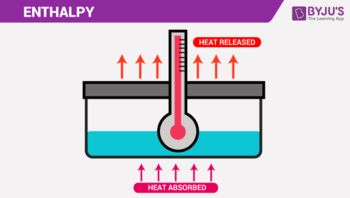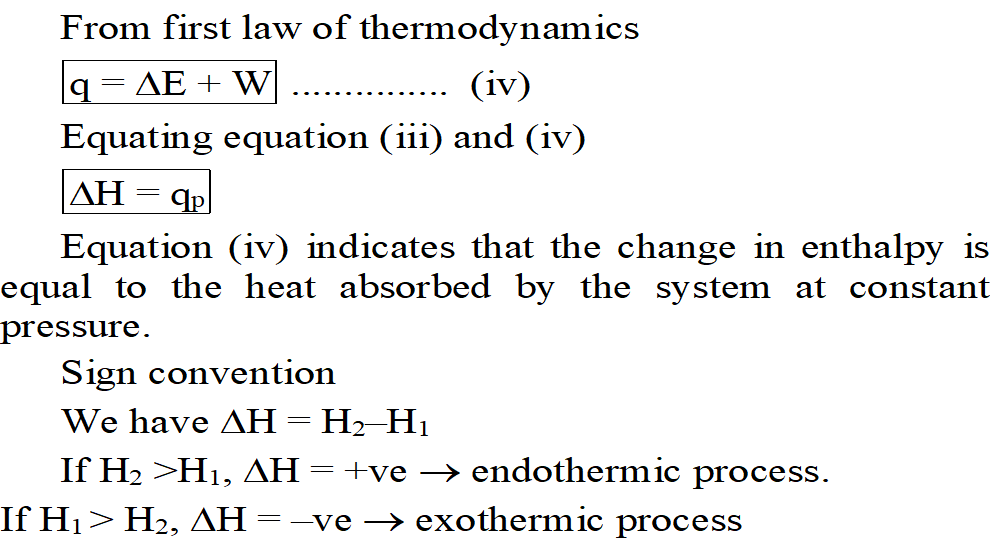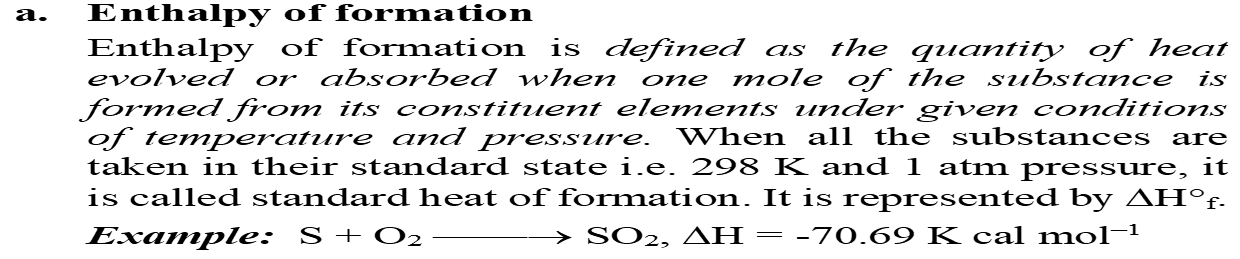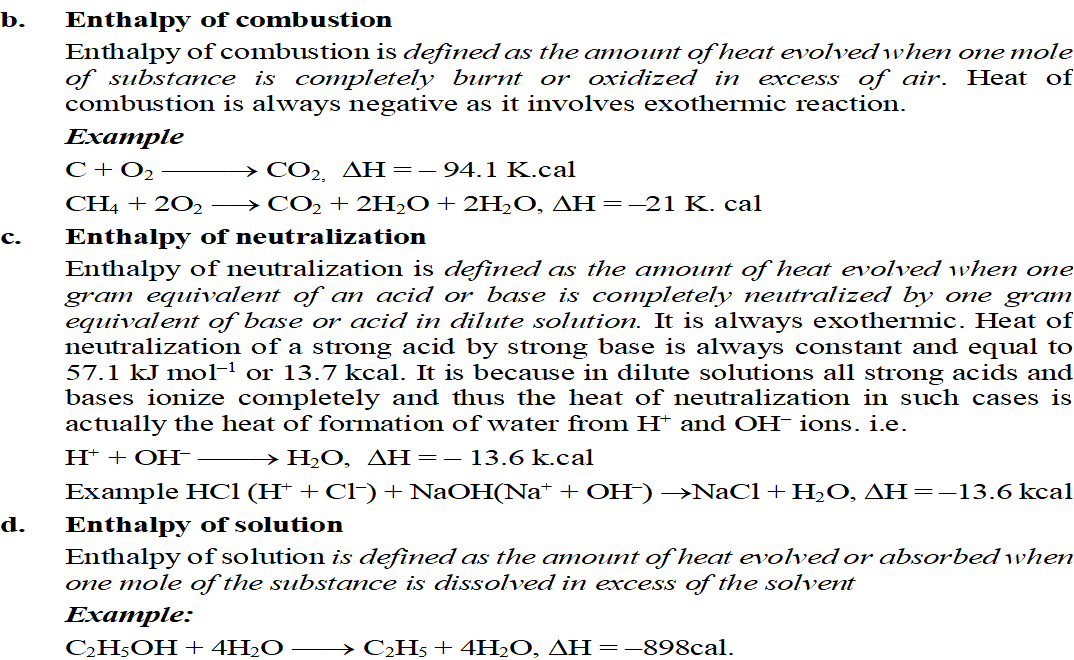EnthalpyEnthalpy

The energy stored in a substance that can be available in the form of heat is called enthalpy or heat constant. It indicated by the symbol H.

Mathematically

H = E + PV ………….(i)

Where E = internal energy

P = Pressure

V = Volume

Enthalpy Change

Enthalpy change is the heat evolved or absorbed at constant pressure. (ΔH = Qp)

Since E, V and P are state function so H is also a state function. The change in enthalpy is calculated by using relation

ΔH = H2 – H1 ……………(ii)

Where H2 = enthalpy of product

H1 = enthalpy of reactant

At constant pressure

ΔH = E2 + PV2 – E1 – PV1

Or, ΔH = E2 – E1 + PV2 – PV1

Or,

 ΔH = ΔE +  PΔVRelation between ΔH, ΔE and Δn

We have

ΔH = ΔE + PΔV

From ideal gas equation.

PV1 = n1Rt ……(i) (at constant temperature and pressure)

PV2 = n2Rt ……(ii)

Subtracting equation (ii) from (i)

PV2 – PV1 = ΔnRT…….(iii)

ΔH = ΔE + ΔnRT

Enthalpy of physical transformation

1.    Enthalpy of fusion:

The enthalpy of fusion of substance is defined as the amount of het required to convert one mole of solid substance into liquid at its melting point. If is indicated by symbol ΔHf.

Example:H2O(s)                                     H2O(l) ΔHfus = 6.6KJ mol-1

2.    Enthalpy of vaporization

Enthalpy of vaporization is defined as the amount of heat required to convert one mole of the liquid into vapor at the boiling point. It is indicated by the symbol ΔHv

Example:H2O(l)                                    H2O(g) ΔHv = 40.6KJmol-1

3.    Enthalpy of sublimation

Enthalpy of sublimation is defined ads the amount of heat required to convert one mole of solid directly into vapour. (It is equal to heat of fusion + heat of vaporization). It is indicated by symbol ΔHs.

Example:I2(s)                                         I2(g) ΔHsub = 62.39KJmol-1

4.    Enthalpy of transition

Enthalpy of transition is defined as the change in enthalpy when one mole of substance changes from one allotropic form to another.

Enthalpy of chemical transformation